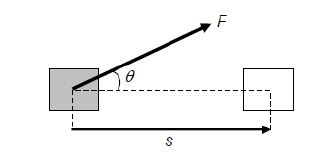Physic Tutorials

# Short note on work, energy and power for Students

Short note on work, energy and power for Students (Cambridge A level and UTME)

Workdone by a force is defined as the product of the force and the distance moved in the direction of the force.

W = F  x s

F is the force

s is the displacement in the direction of the force

w is the workdone

the unit of workdone is joules

Doing work is a way of transferring energy

Joule is defined as the amount of workdone when a force of 1 newton moves a distance of 1 meters in the direction of the force.The horizontal component of the force is Fcosθ

workdone = Fcosθ x s

workdone = Fscosθ

Energy

• Energy can neither be created nor destroyed, but can be converted from one form to another (or others).
• The total amount of energy in any closed system is constant
• There is no change in the total energy of the Universe
• Energy and work are both scalar quantities, and have the unit Joule.

Gas doing work

Gas exert pressure on the wall of their container. if a gas expands, the walls are pushed outwards – the gas has done work on it surrounding.

work done by a gas that is expanding against a constant external pressure: W = p .VPressure = force / area

cross multiply

force = pressure x area

F = pA

workdone = F X S

substitute for the force

workdone = p x A X S

but the quantity A x s is the increase in volume of the gas, which is ∆v

w = p∆v

we assume that pressure p does not change as the gas expands. This will be true if the gas is expanding against the pressure of the atmosphere, which changes only very slowly.

Derive, from the equations of motion, the formula Ek = ½ mv2

Kinetic Energy gained by an object is equal to the work done on that object

potential energy is the energy an object has because of its position or shape

workdone by a net force = change in kinetic energy of the body

recall that                                    v2 = u2 + 2as

make as the subject of the equation                         as = ½ v2 – ½ u2

Multiple both sides by m which is the mas            mas = ½ mv2 – ½ mu2

F=ma , so                                        Fs = ½ mv2 – ½ mu2

I f an object is starting from rest u = 0

Fs = ½ mv2

Ek = ½ mv2

Kinetic energy = energy associated with a moving object

Distinguish between gravitational potential energy, electric potential energy and elastic potential energy

gravitational potential energy : it is the stored energy available to do work due to position of mass in a gravitational field

elastic potential energy is the energy stored in an object which have had their shape changed elastically

G.P.E = mgh

Elastic potential = 1/2 k x^2

show an understanding of the concept of internal energy

Internal energy: is the sum of the random potential and kinetic energies of all the molecules in a body

Internal Energy = Total Potential Energy + Total Random Kinetic Energy

Random Kinetic Energy = Translational Kinetic Energy + Rotational Kinetic Energy

Translational energy is the energy associated with the whole molecule moving in a certain direction.

Rotational energy is the energy associated with the molecule rotation around a certain point.

Potential energy is the energy associated with intermolecular forces

Efficiency = (useful output energy / total input energy) x 100%

Define power as work done per unit time and derive power as the product of force and velocity

Power is defined as the rate of workdone

power = w / t

watt is the unit of power

watt is defined as a rate of working of 1 joule per second

### Bolarinwa Olajire

A lecturer, Educationist, PhD student at FUNAAB, and a Blogger.